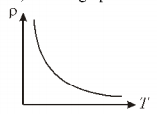# RBSE Solutions For Class 12 Physics Chapter 5: Electric Current | Textbook Important Questions & Answers

The answers are provided to all the questions of Chapter 5 Physics of RBSE Class 12. Students can go through these questions to understand the concepts better and score well in the board examination and entrance examinations for various professional courses. These solutions are provided by the team of experts to provide the best and accurate solutions to the questions. Do check BYJU’S RBSE Class 12 solutions page to get the answers for textbook questions of all subjects.

## Multiple Choice Questions

Q1: The product of resistivity and conductivity of the conductor depends upon

1. The cross-sectional area
2. The temperature
3. The length
4. None of these

Q2: A conducting resistance is connected to the battery and temperature of the conductor decreases by the process of cooling then the value of current will be

1. Increased
2. Decreased
3. Remain constant
4. zero

Q3: The electric power is supplied through the copper wires from one city to another city which is 150 km apart. If the terminal voltage and average resistance per kilometre are 8 volt and 0.5 ohms, respectively then the power loss in a wire is

1. 19.2 W
2. 19.2 kW
3. 19.2 J
4. 12.2 kW

Q4: When the wire is connected with a battery is heated up due to electric current which quantity does not vary

1. Drift velocity
2. Resistivity
3. Resistance
4. Number of free electrons

Q1: From the following graph between V and I calculate the resistance of the resistor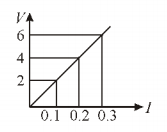Answer: Slope of the line is given by V/I

V/I = 6/0.3 = 20Ω

Q2: Write down the SI unit for current density

Answer: The current density is given by the current per unit area. Therefore, the unit is given by

Ampere/m2

Q3: Write down the relation between conductivity and current density of a conductor

Answer: The relation between conductivity(σ) and current density(J) is given by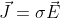Where E is the electric field

Q4: Give two examples of non-ohmic devices

Answer: Diode and electrolytes are examples of non-ohmic devices

Q5: Give the dependence of resistivity on the temperature of a conductor

Answer: The relation between resistivity and temperature is given by

ρt0(1+α(t – tref))

Where ρt = Conductive resistance at temperature T

ρ0 = Conductive resistance at temperature Tref

α =Temperature coefficient of resistance for conductor material

t= Conductor temperature

tref = Reference Temperature

Q6: Write the names of two materials whose resistivity decreases on increasing the temperature

Answer: Germanium and Silicon are examples of materials whose resistivity decreases on increasing the temperature. Germanium and Silicon are semiconductors.

Q7: Write the value of current flowing through a bulb of 40 W 220 V

The power of the bulb is given by P=40 W

Voltage of bulb=220 V

I=P/V=40/220=0.1818 amp

Q1: What will be the value of charge when an electric current flows through a conductor?

Answer: When an electric current flows through a conductor it will not charge. Therefore, the total charge across it will be zero.

Q2: In the given figure, the resistivity of some conductors are ρ1 and ρ2. What is the ratio of ρ1 and ρ2.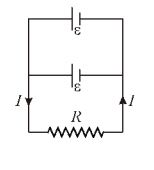Answer: Resistivity of a conductor does not depend on the length and area of cross-section of the material

Therefore ρ1:ρ2 =1:1

Q3: In the given figure, there are two similar cells whose emfs are same and internal resistance are negligible. These cells are connected in parallel. What is the value of current flowing through the resistance R?Answer: In parallel combination, the potential difference will be equal to the emf of the battery

V =є

Current, I=

Therefore I= є/R

Q4: Explain the difference between the terminal voltage and the emf of the cell.

Terminal Velocity: When the cell is in closed circuit i.e. the current is flowing through the cell and the external circuit then the potential difference across its electrodes is called the terminal velocity

Electromotive force (emf): When the cell is in open circuit, then the potential difference across its electrodes is called e.m.f

Q5: Define drift velocity.

Answer: The average velocity with which the free electrons travel across the conductor when an electric field is applied is called drift velocity.

Drift velocity Vd=(-eE/m)τ

e = magnitude of the charge on an electron

m = mass of the electron

E = electric field

τ = average relaxation time

Q6: A resistance wire of 8R is bent in the form of a circle, then what is its equivalent resistance across the ends of a diameter.

When the resistor is folded into the circular form, then for equivalent resistance the circle is divided into two across the diameter, therefore the resistance becomes half

R1=8/2=4Ω

R2=8/2= 4Ω

On connecting them in parallel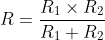R =(4×4)/8 = 2Ω

Q7: When the shape of a conductor is deformed then what is the effect on its resistance and resistivity?

The resistance is inversely proportional to the area of cross-section while resistivity is not affected by the shape change of the conductor.

Q8: Can the terminal voltage of a cell be greater than the emf of the cell?

Yes, When the cell is charging

Vt=є+Ir

Where I= current in the circuit

r = internal resistance of the cell

Vt= terminal velocity

є= emf

## Essay Type Questions

Q1: Derive the relation between the drift velocity and electric field. What is mobility? Explain the dependence of drift velocity and mobility.

Answer: Consider a conductor of length l and the potential difference across the conductor is V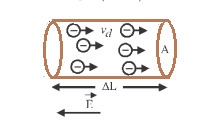Let n be the number of free electrons per unit volume inside the conductor. The area of the cross-section is given by A. If the electric field applied across the conductor the free electrons will move opposite the direction of the electric field. All the free electrons can be assumed to be moving with the same drift velocity Vd. Let us consider a small length ΔL. The number of charge carriers in this small element is nAΔL.

The net charge on these charge carriers will be ΔQ= (nAΔL)e

The time taken by the charges to cross this small element of length(ΔL) is

Δt=ΔL/Vd

The value of the current is given by I=ΔQ/Δt

I=(nAΔL)eVd/ΔL

Therefore, the relation between the current and drift velocity is given as

I=nAeVd

Mobility: Mobility is the drift velocity per unit electric field. It is denoted by μ.

μ=Vd/E

The S.I unit of mobility is m2/(V⋅s)

Q2: Establish the relation between resistance and resistivity. How does the resistivity depend on the temperature? Describe conductors, insulators and semiconductors on the bases of resistivity.

Relation between Resistance and Resistivity

The resistance depends on the length L and area of cross-section A of the resistor

R∝ L/A

Or R=ρL/A

Where ρ is the specific resistance or resistivity of the material of the conductor. If resistivity depends on the characteristics of the conductor. It does not depend on the length or cross-sectional area of the conductor.

Effect of Temperature on Resistivity

The resistivity is given by

ρ = m/ne2τ

Where m = mass of the electron

e=charge of electron

n=number free electron per unit volume

τ =relaxation time

The temperature will not affect the mass of the electron (m), the charge of the electron (e) and the number of electrons (e). Let’s find out how temperature depends on relaxation time (τ).

The relaxation time τ = λ/Vrms

Where λ is the mean free path

Vrms is the root mean square velocity of electrons

When there is an increase in temperature the vibrational energy of atoms of the conductor increases and hence there is an increase in amplitude in vibration. This causes the mean free path λ to decrease. There is an increase in Vrms as the temperature increases. Due to the combined effect of λ and Vrms the value of relaxation time decreases

From the equation of resistivity, we know as the relaxation time decreases with temperature there will be an increase in resistivity.

(i) For conductors

The change in resistivity with an increase in temperature is given by the equation

ρt0(1+αt)

Whereρt is the resistivity at a temperature t

ρ0 is the resistivity at a temperature 0 degree celsius

α = linear temperature coefficient of resistivity

The above equation can be rewritten as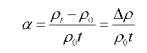The graphical representation of the change in resistivity at low temperature is a curve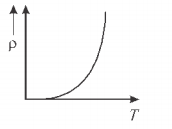At temperature dependence of resistivity at higher temperature is given by the graph below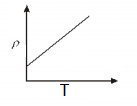(ii) For insulators

The resistivity of insulating material decreases exponentially with increasing temperature and vice-versa. Mathematical expression for the variation of resistivity with temperature for an insulator given by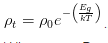Where k is the Boltzmann constant

T is the temperature in kelvin

Eg is the energy gap between the conduction band

(ii)For semiconductors

The resistivity of semiconductor material decreases exponentially with increasing temperature. The graph below shows the variation of resistivity with temperature for a semiconductor.